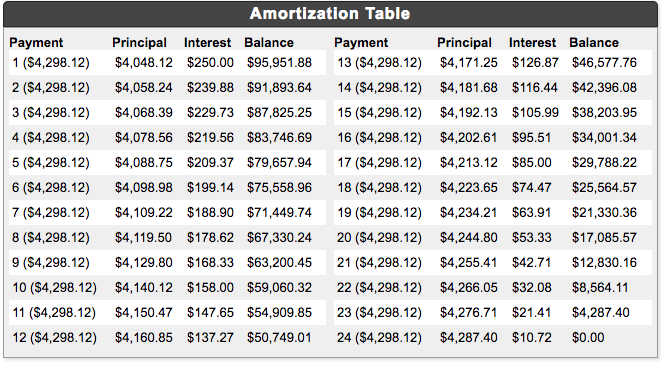## Amortization Formulas

2017 March 30

Derive the formula for the monthly payment in an amortization loan schedule.

One interesting application of the iterated function is the calculation of formulas for an amortization schedule used to pay off loans. In such a plan, a monthly payment is made for the same amount each month for some number of payments. Though each payment is for the same amount, each payment is divided up into an interest payment and a principal payment. The portion of each payment that is paid as interest is the monthly interest rate (or $\frac{1}{12}$ of the annual interest rate) times the current balance (amount owed on the loan), and the rest of the payment is the principal payment, going towards paying down the balance. With each payment, the balance decreases, and less interest is paid at the next payment, meaning that the balance is paid down more and more quickly. Here is an example:

Suppose you take out a loan of $\$100,000$at an annual interest rate of$3\%$to be paid off over a$2$year period. This means that you will make a total of$24$monthly payments at a monthly interest rate of$0.25\%$, or$0.0025$. In this case, the monthly payment will be about$\$4,298.12$. For the first payment, you pay $\$4,298.12$as with every other payment, and$0.0025*\$100,000=\$250$of this payment goes towards interest. The rest of the payment is the principal payment, which is about$\$4298.12-\$250=\$4048.12$, reducing the balance to $\$95,951.88$. At the next payment, the part of the payment going towards interest is a little bit less, since the balance decreased, and more of the payment is principal. Here is a table showing all$24$of the payments:Given the initial balance, the number of payments, and the monthly interest amount, finding the monthly payment can be a tricky task. However, we can set up an iterated function to calculate it. First, we assign variables to the givens and the unknowns. Our givens are$L$, the loan amount;$m$, the monthly interest rate; and$n$, the number of payments. Our unknowns are$p$, the monthly payment;$i_k$, the interest amount at the kth payment;$l_k$be the interest amount at the kth payment; and$B_k$, the balance after the kth payment. We already know one thing about the relationship between$i_k$and$B_k$; namely, that because the interest of the kth payment is the monthly interest times rate times the current balance before the payment is made. We also know that because whatever part of a payment not interest is the principal part of the payment. Also, because whatever is not interest goes towards the loan, We can now substitute$p-i_k$for$l_k$and we get We also can substitute$mB_{k-1}$for$i_k$, so We have turned$B_k$into a sequence. In order to finish defining the sequence, we need only one term of the sequence. We already know that before any payments, the balance is$L$, so we can let$B_0=L$. Then we can define$B_k$as where However, since$B_0=L$, then and by our formula for the nth iteration of functions of the form$f(x)=cx+d$, and But this is still in terms of$p$, which is an unknown as of yet. However, we can solve for$p$because we know another value of$B_k$. Since, after the last payment, the balance will be$0$, we can say that$B_n=0$. We can substitute and solve for$p$: Ok, we've found the monthly payment. But those loan companies are tricky little buggers. Say we also want to find out the total amount that we'll end up paying them, or better yet, the total amount of interest that we end up paying. The total amount paid is an easy quantity to find - it is simply the number of monthly payments times the monthly payment, or And the total amount of interest paid is the difference between the total amount paid and the loan amount, which is To summarize, if one is using an amortization schedule to pay off a loan of$L$with a monthly interest rate of$m$over the course of$n$payments, the monthly payment$p\$ is given by

and the total amount of interest paid is